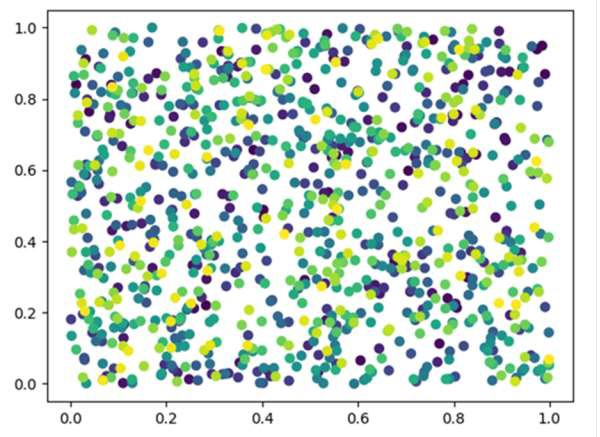# Scatter plot and Color mapping in Python

We can create a scatter plot using the scatter() method and we can set the color for every data point.

## Steps

• Create random values (for x and y) in a given shape, using np.random.rand() method.

• Create a scatter plot of *y* vs. *x* with varying marker size and/or color, using the scatter method where color range would be in the range of (0, 1000).

• Show the figure using plt.show().

## Example

import matplotlib.pyplot as plt
import numpy as np

x = np.random.rand(1000)
y = np.random.rand(1000)

plt.scatter(x, y, c=[i for i in range(1000)])
plt.show()

## OutputUpdated on: 16-Mar-2021

491 Views